## WHY GUIDELINES FOR BENEFICIAL USE OF SLUDGES IN AGRICULTURE ARE DIFFERENT AND ESTIMATES OF ALTERNATIVES

D. R. Bouldin

Soil, Crop, and Atmospheric Sciences, Cornell University, Ithaca NY, 14850

Published in the Soil Science Society of North Carolina Proceedings, Vol. XXXX (1997)

### ABSTRACT

The metals zinc and copper, among others elements, are essential to plant and animal life yet depress crop yields if added in excess. Maximum cumulative loads are estimates of the amount of a metal at which the depression in crop yields become unacceptable. The implications of such a limit is that a) the effects of previous additions persist and are additive to current applications and b) if cumulative additions lead to yield reductions, these yield reductions may persist even if additions of metals via sludges are stopped.

Maximum cumulative loads of metals from application of sewage sludges to agricultural land differ by an order of magnitude among groups developing them. All use the same data yet arrive at different end points. In the following discussion the reasons for these differences are illustrated and several examples of alternative risk-based maximum cumulative loads are derived. As the probability of yield reductions approach zero, the maximum cumulative loads are 10 to 100 times less than the EPA 503 cumulative loads. These alternatives give farmers and others a perspective on how to develop a maximum cumulative load for their own situation if they are planning to landspread sludges and products containing sludges.

### INTRODUCTION

An essential component of any program for land spreading of sewage sludges is the setting of maximum cumulative loads. The technical term "cumulative load" is the sum over years of the amounts of a substance added. The "maximum cumulative load" is a selected limit such that further applications of sludges should cease once this limit is reached. Several implications of this limit are listed below.

1) One implication of this limit is that the risk to decreased soil productivity is acceptable so long as it is not exceeded. The idea of risk must be introduced because the experimental data on which to base estimates of maximum cumulative loads is limited and hence we cannot define with certainty a cumulative load which will result in a given (or no) yield decline.

2)An additional implication is that annual additions are less important than the cumulative amount added. This implication is a consequence of the common observation that the elements listed in the table have residual effects that carry over from year to year and that these effects accumulate. The point at which accumulated metals cause decreases in yield depends on the characteristics of the soils, crops, and sludges and hence maximum cumulative loads depend on these same variables.

3) Another implication of the residual effects is that any adverse effects may persist even after the application of sludges has ceased. As a consequence, amelioration of adverse effects may be difficult and impractical.

4)Another implication is that initially applications may increase crop yields because of plant response to nutrients and organic matter in the sludge but eventually the accumulation of any of several elements will reduce yields because of toxicity. This means that users of sludges and products containing sludges need to be aware that eventually soil productivity will be decreased. In addition, the plant uptake of some metals increases as the soil pH decreases which means that while the pH is 6.0 or above, problems will not be evident but if the pH drops the metals may become toxic.

Table 1. Comparison of maximum cumulative loads of 4 metals, median content in 3045 US soils and content in a crop of corn silage

 Element Maximum Cumulative Load (kg/ha) Median US soil (kg/ha) + Corn Silage (kg/ha)++ EPA* NEC-28** Ontario*** Cd 39 24.5 1.6 0.6 0.001 Cu 1500 25-150 210 60 0.01 Ni 420 10-60 32 60 0.005 Zn 2800 50-300 330 160 0.1

* EPA. 1993
** NEC-28.1985
*** Ontario. 1992
+Holmgren et. al. 1993. Kg/ha @ bulk density =1, depth = 30 cm
++ Kelling et. al. 1977

Table 1 lists the maximum cumulative load of several elements in columns 2, 3 and 4. Those in column 2 were developed by EPA for the whole US. Those in column 3 were developed by 26 northeastern US soil and crop scientists because they believed the soil and crop conditions peculiar to their region were sufficient to warrant lower loads than those for the US as a whole. Note that they are about 1/10 of the EPA maximum cumulative loads and give ranges based on soil texture. Those in column 4 were developed by the Province of Ontario for their conditions. They are similar to the NEC-28 maximum cumulative loads. The European Community has adopted regulations/guidelines which are similar to the NEC-28 and Ontario maximum cumulative loads.

Columns 5 and 6 are included to provide a frame of reference. Column 5 is the median content of 3045 samples of soil from the US. In comparison, the EPA recommended cumulative loads would increase the soil content by a factor of 65 for Cd, 25 for Cu and 18 for Zn and 7 for NI. The last column lists the amount removed in a conventional crop of corn silage; it is clearly several thousand times less than the EPA recommended cumulative load and illustrates that crop removal is not a major factor in reducing the cumulative loads.

The question we address is why these estimates of maximum cumulative loads differ so much among groups. The following discussion will describe how EPA developed their regulations for Zn . The substance of that discussion is to illustrate that maximum cumulative loads depend on the selected risk of reduced crop yields. We illustrate that this process is both difficult and arbitrary.

### EPA PROCEDURES AND ALTERNATIVES

A brief description of the procedure used by EPA follows. First step: the relationship between yield and tissue content of Zn was established. Using these data, an acceptable level of yield reduction was selected and the corresponding Zn content of the tissue was selected. Second step: the relationship between Zn tissue content and the amount of Zn added in sewage sludges in other experiments was developed. Third step: In "approach 1" (EPA 1992, pp. 5-204) they calculated the probability that the selected tissue content would be observed for given applications of metals in sludge. The basis for these calculations is also described in Chang et. al. (1992). In "approach 2" (EPA 1992, pp. 5-205) the tissue content selected in the first step was substituted into the relationship developed in the second step and the result is the maximum cumulative load. These three steps are illustrated in the following paragraphs.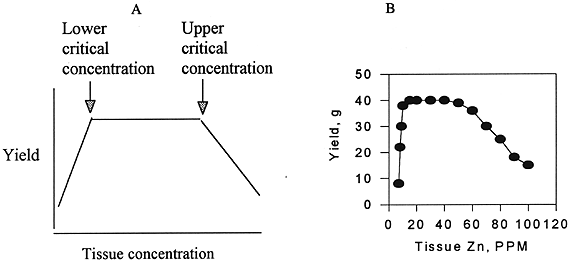Figure 1. 1 A. conceptual diagram of crop response to increasing tissue content. 1 B. Data for sorghum (Ohki. 1984).

First step: A conceptual frame work for the relationship between yield of a crop and the content of essential elements is illustrated in Figure 1A. The left hand side of figure 1A shows the yield while the bottom scale shows the elemental content of the crop. Starting with severe deficiencies on the left hand side, the yields increase but soon reach a plateau where the yields remain constant as the content increases until a point at which yields begin to decrease because excessive metals are interfering with some essential (often unknown) process. Note that the yields decrease rather slowly in the toxic range. The lowest content on the yield plateau will be referred to as the "lower critical level" and the point at which the yields begin to decrease will be referred to as the "upper critical level". Figure 1B illustrates the above concept with sorghum which shows the whole relationship from deficiency to toxicity.

Now we look at the data for the second step. Shown in figure 2 is the relationship between Zn in corn stover and cumulative amount of Zn applied in sludge. This data is taken from one of the best experiments with cumulative additions of sludge (Hinesly and Hansen, 1983). Beginning in 1969, applications of four rates of sludge (including none) were applied annually to continuous corn plots. From 1971 through 1977 the leaves was analyzed for metal content and the data shown in figure 2 is the content in the stover for the treatments for each of the 6 years. Based on these data the Zn content of the corn stover increased about 0.14 ppm for each kg/ha of Zn applied in the sludge.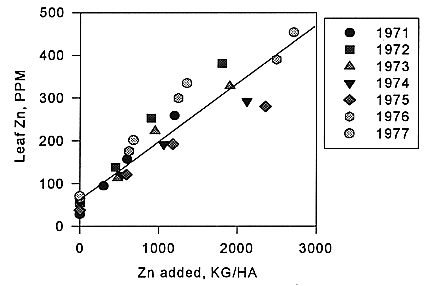Figure 2. Zn content of leaves of field grown corn plotted against the cumulative amount of Zn added in sewage sludges. Points are experimental observations, line is regression: Zn in leaves = 69 + 0.14* cumulative Zn, n = 26, R*R = 0.88.

It also illustrates nicely the cumulative effects of Zn additions. Further statistical analysis of the data does not support the hypothesis that current additions of sludge Zn are more susceptible to crop uptake than residual effects of previous additions.

As might be expected the increase in Zn in crop tissue for the same addition of Zn is very different among experiments. This will be illustrated more fully in later discussion.

In step three, EPA combined the foregoing data to produce estimates of the maximum cumulative loads. EPA used two "approaches". In approach one they estimated the Zn content in corn tissue which gave a 50% reduction in yield to be 1975 ppm and then calculated the probability that this content of Zn would be obtained based on data from field experiments with corn in their data base (EPA 1992, appendix F). The results of this analysis is that the maximum cumulative load for Zn is over 3500 kg/ ha and that so long as the cumulative load was less than this, the probability of observing 1975 ppm Zn in corn tissue was less than 0.01%.

In a publication which appears to be the basis for the EPA analysis described above, other levels of yield reduction were tabulated, the corresponding tissue Zn concentrations, and the probability of observing this concentration is shown in Table 2 which is derived from Chang et. al. (1992). For example if an 8% yield reduction is selected, Zn additions of 200-500 kg/ha could be expected to reduce yield by 8% about 10 out of 100 trials.

Table 2. Probability of Zn in corn tissue grown on sludge-treated soils exceeding the estimated content associated with specified yield reductions (Chang et. al. 1992). The last column in the table is essentially the same as that is EPA (1992 p. 5-206).

 Zn loading range Tolerance Threshold (mg/kg )* (kg/ha) 250 500 1000 1975 0-50 <0.0001 <0.0001 <0.0001 <0.0001 50-200 0.02 0.007 <0.0001 <0.0001 200-500 0.10 0.01 <0.0001 <0.0001 500-1000 0.26 0.05 0.003 <0.0001 1000-2500 0.30 0.025 0.04 0.001 2500-3500 0.35 0.04 0.001 <0.0001

*Tolerance Thresholds 250, 500, 1000, 1975 correspond to estimated yield depressions of 8,10, 25 and 50% respectively

Figure 3. 3A. Sample of data relating relative yield of corn forage to tissue Zn content taken from EPA 1992, appendix F and Chang et. al. 1993. 3B. Relationship between crop tissue content and cumulative amount of Zn applied in sludge

Now we illustrate an alternative procedure with data on corn forage which illustrates that the maximum cumulative load depends on how the data is selected t averaged. Shown in figure 3A are relative yields of corn forage plotted against the Zn content of the forage for several experiments; this will be used to define acceptable contents in forage. In figure 3B we have plotted the Zn content in corn forage from other experiments against the amount of Zn added in sewage sludge in field experiments; this will be used to establish limits on the amount of sludgeborne Zn which can be applied consistent with the limits on Zn in forage from the previous step. In each case, the symbols connected by lines represent the results with a specific variety and soil. Note that the data is restricted to corn forage.

Examination of these 2 figures immediately leads to questions about how to implement the three steps. Figure 3A shows how yields are reduced by excessive Zn in the tissue but that the relationship between yield reduction and tissue Zn varies considerable among the several sets of data. Similarly Figure 3B shows that the increase in Zn in the tissue for a given addition of sludge borne Zn differs among experiments. In some cases there was a large increase in Zn content of the crop for a given addition of Zn in sewage sludge while in other cases there was a relatively smaller increase in Zn content of tissues for a similar addition of sludge borne Zn.

Important choices must be made about how to use this data. For example: a) To implement the first step a choice of acceptable yield loss must be made. Should it be: none?, 10%?, 20%?, 50%. Next a choice about which experimental data to use. The most protective of soil productivity would be the "worst observed case"; that is, the data in Figure 3A which shows the most yield reduction for the lowest tissue Zn content and in figure 3B the largest increase in Zn tissue content for a given additions of Zn. Another alternative would be to use the averages or medians of all observations. The implications of these several choices are illustrated graphically in the following scenarios.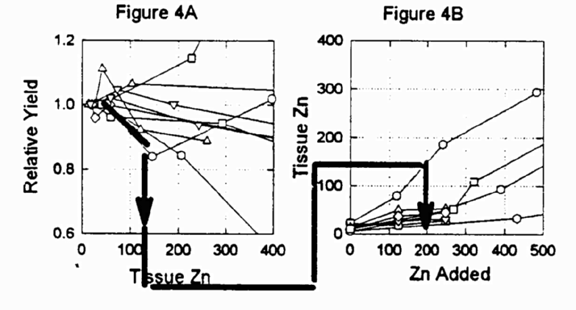Figure 4. An illustration of scenario one with bold lines linking the two figures. Same data as in figure 3 except scale changed to facilitate interpretation.

Scenario one.

a) The acceptable yield reduction is selected to be 10%

b) The relationship between yield reduction and Zn in the forage is most protective (Figure 4A)

c) The relationship between Zn in forage and applied Zn is most protective (Figure 4B)

This is the same data as in Figures 3A and 3B except that the scales on the axes have been changed to make the graphs more amenable to graphical interpretation. The bold line in Figure 4A is selected as the relationship between yield reduction and tissue zinc in the corn forage and in figure 4B as the relationship between tissue zinc and metal added as sludge; the position of these two lines is based on the conditions of the scenario. The arrows in each case leading to the X-axis is the consequence of the scenario. In this case, tissue zinc content, of 120 results in 10 % yield reduction and approximately 200 kg/ha of zinc added in a sludge resulted in this tissue zinc (figure 4B). Hence the maximum cumulative load would be 200 kg/ha under this scenario.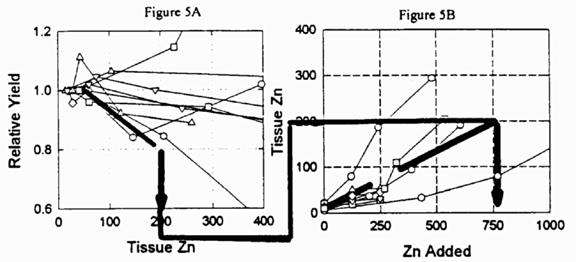Figure 5. An illustration of scenario two with bold lines linking the two figures. Same data as in Figure 3 except scale changed to facilitate interpretation.

Scenario two.

a). The acceptable yield reduction is selected to be 20%.

b) The relationship between yield reduction and Zn in tissue is the most protective (Figure 5A).

c) The relationship between Zn in crop and applied Zn is approximately the median (Figure 5B). Thus the difference from Scenario 1 is the 20 % yield reduction instead of 10% and the use of the median vs. most protective data in determining the amount of Zn added which will result in the acceptable level of tissue Zn (step 3) Under this scenario the maximum cumulative load is about 750 kg/ha, more than three times the level determined under Scenario 1.

Scenario three.

a) The acceptable yield reduction is selected to be 50%.

b) The relationship between yield reduction and Zn in tissue is approximately the median (not most protective) (Figure 6A, next page).

c) The relationship between Zn in the tissue and applied Zn is approximately the median (not most protective) (Figure 6B, next page).

Thus the differences from Scenario 2 is the use of 50% yield reduction and of median vs. most protective data in determining Zn in tissue which results in 50% yield reduction (step 2). Under this scenario the maximum cumulative load is about 2800 kg/ha, the EPA maximum cumulative limit.

The objective of the above exercise is to illustrate the maximum cumulative load depends upon what are considered to be acceptable yield reductions and safety factors. Using the same data, the choices led to the following maximum cumulative loads:

Scenario 1. 200 kg/ha

Scenario 2. 750 kg/ha

Scenario 3. 2800 kg/ha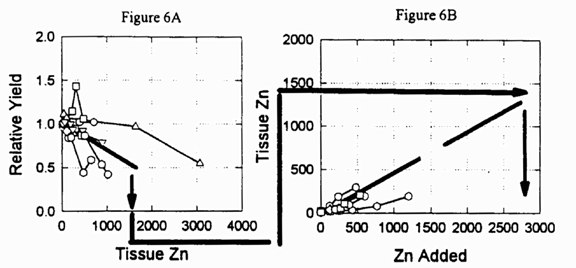Figure 6. An illustration of scenario three with bold lines linking the two figures. Same data as figure 3 except scales changed to facilitate interpretation.

The choices made in Scenario 1 approximate those used by the NEC-28 committee (column 3, Table 1) in determining their maximum cumulative load while those in Scenario 3 are approximately those used by EPA in determining the 503 regulations (column 2, table 1).

As pointed out by EPA (1992, pp 5-198), corn is one of the least sensitive crops to excessive Zn. According to EPA lettuce is among the more sensitive crops (EPA, 1992, pp 5-198). Accordingly for "approach 2" EPA adopted the procedure illustrated in Figure 7 as a way to estimate maximum cumulative loads for sensitive crops.

 Critical concentration Zn minus Zn in crop w/o sludge divided by Uptake coefficient equal Maximum cumulative load 400 PPM 47 PPM 0.125 PPM/KG 2800 KG/HA

Figure 7. Diagram of the EPA "approach 2" (EPA 1992, p. 5-205)

In this approach, the tissue concentration is set at the "upper critical level" (the point at which the yield begins to decrease as illustrated in Figure 1). Next the content of the crop without sludge is subtracted from this number to derive the amount by which the Zn content of the tissue can be increased to just bring the concentration to the upper critical level. Finally this difference is divided by the increase in Zn content in the crop per unit of added sludge Zn.

As shown in Fig 7, EPA analyzed the data for lettuce; by averaging techniques they set the critical upper concentration of Zn to be 400 ppm, the content in the absence of sludge to be 47 ppm and the uptake coefficient to be 0.125; thus the maximum cumulative loading = (400-47)/0.125 = 2800 yielding a maximum cumulative load of 2800 kg/ha, which is a bit less than that found in their approach 1.

Shown in Figure 8 (next page) is a frequency distribution of critical upper concentrations based on a literature search for all crops by R. M. Macnicol and P. H. T. Beckett (1985). Most of the references cited by Macnicol and Beckett can also be found in the EPA data base (EPA. 1992. appendix table E). As can be seen from this figure there are very large differences among crops /experiments.

Shown in Figure 9 are the uptake coefficients for Zinc (increase in crop content per unit of applied metal), based on the EPA data base (1992, appendix C) for corn, lettuce and cabbage. These become the divisors in the calculations diagrammed in Figure 7. Again there is a very wide range of uptake coefficients and there is no clear way to decide which are most appropriate to use in calculations of maximum cumulative loads.

Several alternative analyses are described in the following. In general, the data in figures 8 and 9 are independent so they may be combined as shown in Figure 10. In the analysis, the lowest maximum cumulative loads will be combinations of small critical concentrations and large uptake coefficients. The first line in the table is derived by combining the critical concentration which is the lowest 10% of the observations shown in the figure on the left (most sensitive crops) with the uptake coefficient (figure on the right) which is not exceeded by 10% of the observations. This combination leads to probability of 10% of 10% or 1% of a yield reduction According to Figure 8, about 10% of the observations were less than 100 ppm. According to Figure 9 about 10 % of the uptake coefficients were greater than 0.5 ppm/kg. Setting the Zn content of crops without sludge at 20 ppm, the maximum cumulative load becomes (100-20)/0.5= 160 kg/ha.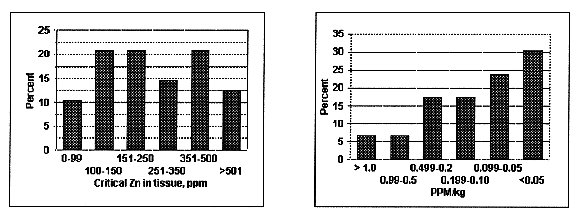Figure 8. Frequency distribution of 48 estimates of the upper critical concentration of Zn. Arithmetic mean = 293, Geometric mean = 231, Median = 250. Sources: Macnicol and Beckett .1985. Rashid and Fox. 1992. Carrol and Lonergan 1968. Ibekwe d al. 1996. Figure 9. Frequency distribution of 46 observations of uptake coefficients of Zn for corn, lettuce and cabbage Arithmetic mean = O.25, Geometric mean = O.092, Median = 0.093

 Critical concentration Zn minus Zn in crop w/o sludge divided by Uptake coefficient equal Maximum cumulative load

 Probability of yield decrease less than Critical concentration PPM Crop Zn w/o sludge PPM Uptake coefficient PPM/KG Maximum load KG/HA 1% 100 20 0.5 160 10% 150 20 0.2 650 25% 250 20 0.093 2500

Figure 10. combination of upper critical Zn in tissue with uptake coefficients to produce estimates of maximum cumulative loads.

The expectation is that if a similar number of measurements were made in only 1% of the measurements would there be a yield decrease. The second line in the table is derived by combining about 33% with 33% to obtain a 10% probability and the medians (the median is the number such that half of the observations are larger than the median and half are smaller) were combined to obtain a 25% probability.

Next we examine the consequences of different cumulative loads on the yield decreases which may occur with crops differing in sensitivity to Zn toxicity. This entails combining the relationships between a) the cumulative load b) the uptake coefficient and c) yield decrease as a function of Zn in tissue.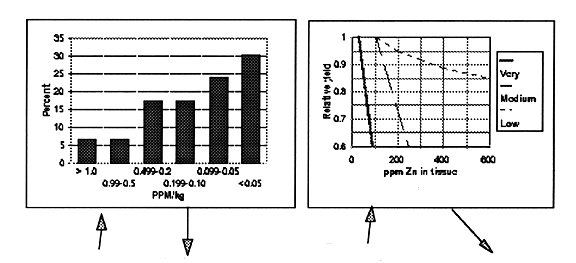Cumulative load Zn, KG/Ha Frequency of larger uptake coefficient Uptake coefficient Expected concentration in tissue w/o sludge Expected concentration in tissue with sludge Relative yield for Relative sensitivity Very Medium Low A B C D =(A*C+D) F G H 60 5% 1 20 80 0.7 1 1 60 10% 0.5 20 50 0.9 1 1 60 50% 0.093 20 25 1 1 1 120 5% 1 20 140 <0 6 0.9 1 120 10% 0.5 20 80 0.7 1 1 120 50% 0.093 20 32 1 1 1 550 5% 1 20 570 <0.6 <0.6 0.85 550 10% 0.5 20 298 <0.6 <0.6 0.93 550 50% 0.093 20 71 0.5 1 1 2800 5% 1 20 2800 0 0 <0.5 2800 10% 0.5 20 1400 0 0 0.6 2800 50% 0.093 20 280 0 <0.6 0.92

Figure 11. Estimates of yields for example cumulative loads with different expected uptake coefficients for crops differing in sensitivity.

Shown in figure 11 is the relationship between relative yield and Zn content of the tissue for three examples taken from the literature. The most sensitive example was obtained with alfalfa in a nutrient culture study (Ibekwe, A. M., J. S. Angle, R. L. Chaney and P. van Berkum. 1996). The medium sensitive example was obtained from a field experiment with bushbeans (Giordano, P.M., J. J Mortvedt and D. A. Mays. 1975). In this case the variables are pod yield as a function of Zn content of vines. The third, and least sensitive example, is corn. This is a plot of the data in the column headings in table 2. It is consistent with field data as will be shown in a later discussion. The variables in this case are yields of corn forage and content of Zn in stover. Based on later discussion it also appears to be the relationship between grain yield and leaf Zn content.

The remainder of Figure 11 illustrates the procedure and results of estimating the yield decreases with different loadings for the different sensitivities. The procedure was as follows. Example cumulative loads are listed in the first column of the table below the graphs in Figure 11. In the second column of the table, example frequencies of larger uptake coefficients is listed. The third column in the table is the uptake coefficient read from the figure on the left, which is not exceeded with the frequency shown in the second column of the table. For example, in the figure on the left, about 5% of the uptake coefficients were greater than 1 or we would not expect the uptake coefficient to be greater than one more than 5% of the time. Next the tissue content without sludge is listed; this is based on unpublished data from NY for corn stover and alfalfa hay. Next the product of the cumulative load and uptake coefficient plus the Zn in the crop without sludge is added and listed in the 5th column of the table. This is the amount of Zn which is expected in the crop tissue and the relative yields can then be estimated from the figure on the right and entered in the last column of the table.

The data in Figure 11 illustrate that with the most sensitive crops catastrophic yield reductions are predicted at EPA cumulative loads. Economically significant yield reductions are predicted at 550 kg/ha Zn (20% of the EPA cumulative load) and even at 5% of the EPA cumulative load, economically yield decreases may be expected occasionally.

### ANALYSIS OF ONE LONG TERM FIELD EXPERIMENT WITH SLUDGE

Probably the best and most complete long term field experiments with sludge were run by Dr. Tom Hinesly and associates in Illinois( Hinesly T. D. and L. G. Hansen. 1983. and Hinesly T D, L G Hansen and D J Bray. 1984). These experiments are about 1/3 of the EPA data base on Zinc toxicity (EPA 1992, Appendix F). Three experiments were started in 1969 which are referred to as "lysimeter", "continuous corn" and "continuous soybeans". On some plots sludge applications were continued through 1980 while on others sludge treatments were terminated at some time during the course of the experiment. Several crop sequences were followed on some plots. The most common treatments were 3 non-zero rates of sludge (1/4, 1/2 and max.) and a fertilized check (no sludge). In the following, only the years and plots in which corn was grown are analyzed. Yields were determined and plant parts (leaf, stover and grain) were analyzed for elemental content beginning in 1970. The sludge was not dewatered and contained 3 to 4 % dry matter. This sludge was added in increments of about 2.5 cm several times during the growing season as furrow irritations with the result that the amount of water added in the sludge treatments was a significant addition to rainfall.

We begin with the continuous corn experiment. In this case the crop was corn and the treatments were continued through 1980. However the check plots did not receive any additional water and hence the question arises about how to interpret the data: how much influence did the additional water from the liquid sludge applications have on the results and could it have overshadowed any negative influence of the sludge on yields? The following describes an analysis of this question.

First the difference in yields between the plots receiving the maximum sludge treatment and the plots receiving no sludge was calculated and will be referred to "max-0."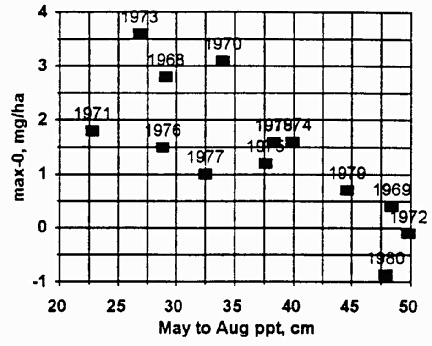Figure 12. Yields on maximum sludge treatments minus yields on non-sludge treatments plotted against precipitation from May through August (Hinesly et al 1983).

Next the max-0 was plotted against the rainfall from May through August and the results are shown in Figure 12. This clearly shows that a) the yields in the sludge treated plots usually out yielded the non-sludge treated plots and b) this difference decreases as the amount of growing season rainfall increases. The latter observation suggests that the water added with the sludge had an important influence on yields which decreased as growing season precipitation increased. To investigate the hypothesis that yields were decreasing as the Zn content of the leaves was increasing, multiple regressions of the form

max-0 = a + b* rainfall during season + c* PPM Zn in the corn leaves

where a, b, and c are constants determined by the regression analysis. The foregoing analysis combines the 3 non-zero rates of sludge over 10 years into one "package". As was expected, max-0 decreased as both rainfall increased and Zn increased. Next these equations were used to calculate the value of max-0 with water set at maximum value and different values of Zn in leaves (in effect this adjusts the yields on the non-sludge treated plots to those expected if the water had been equalized). The results are shown in Figure 13 (next page). The calculated yields do decline as the leaf Zn increases. The net effect of this analysis is to indicate that both water and Zn were influencing yields. Furthermore, yield depression began when the Zn in the leaves exceeded about 100 PPM.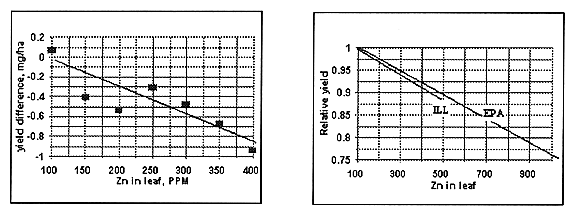Figure 13. Calculated yield difference (sludge treated minus non-treated) in metric tons/ha plotted against Zn in leaves. Figure 14. Relative yield of corn plotted against Zn in leaf. Line labeled "EPA" based on corn forage, Table 4 this ms and line labeled "ILL" based on Figure 13.

Another interesting comparison is shown in Figure 14 where the relative yields of corn are plotted against Zn content of the leaves. The line labeled "EPA" is taken from Table 2 and presumably is the relationship used by EPA to arrive at the value of 1975 ppm Zn in the corn tissue which corresponds to 50% yield reduction. The other line labeled "Ill" is the data described above. This is a remarkable agreement between the EPA data, primarily based on greenhouse pot studies and immature corn, and the field data with corn grain yields described above.

In the lysimeter experiment, there were three "soils". One was the on-site silt loam soil, referred to as "Blount", another was the Blount with surface 30 cm replaced with surface 30 cm of Elliott silt loam, referred to as "Elliott" and a third was Blount with surface 1.5 meter replaced with Plainfield loamy sand, referred to as "Plainfield". In this experiment the plot not receiving sludge received an equivalent amount of water as that applied to the "max" plots. In this case the analysis examined the hypothesis: the yields on non-sludge treated plots were equal to the max. sludge treated plots. This hypothesis was tested by counting the number of cases in which the max sludge treated plots yielded less than the untreated plots. Out of 24 comparisons, in 17 cases the sludge treated plots yielded less than the non- sludge treated plots. If the hypothesis is true this observation has a probability of less than 5%. Thus we reject the hypothesis that the yields in the non-sludge treated plots equal those in sludge treated plots and conclude that indeed the sludge treatments are reducing corn yields. In 21 out of the 24 comparisons, the leaf samples from the sludge treated plots contained more than 100 PPM Zn.

In some plots, sludge applications were terminated in 1974 and in others in 1976. In 1979 and 1980 fertilizer N was applied to all of these plots and the yields were determined. In 12 of 18 comparisons the non-sludge treated plots out yielded the sludge treated plots. In all cases where the corn leaf Zn was greater than 100 ppm on the sludge treated plots, the non-sludge treated plots out yielded the sludge treated plots.

The inference to be drawn from the data is that when the leaf content of field corn exceeds 100 ppm Zn, yield reductions may be expected. In the experiments in Illinois the leaf content of Zn was above 100 ppm once a total of 200 kg/ha of Zn from sludge had been added (Figure 9); this is about 7% of the EPA maximum cumulative limit for Zn.

Would a farmer be able to recognize that his yields are being reduced because of what is presumably Zn toxicity? The answer is probably not until the yield decrease was on the order of 10 %. In most field experiments, the variation among plots is large enough so that yield differences on the order of 10% are not STATISTICALLY significant. However, yield losses greater than zero are ECONOMICALLY significant.

In the "continuous soy bean" experiments the yields with the maximum sludge treated plots decreased by about 60 % the 4th year after the experiments were begun. The reason for this decrease in yield was attributed to "phosphorus toxicity" and not to Zn, Cd, Cu or Ni. We have not analyzed this data further but in our opinion the yield decrease could have been the result of any of several other causes.

This analysis of the Hinesly data on corn yields the following conclusions:

a) Once the Zn in corn leaves exceeds 100 ppm yield reductions were observed. This is consistent with the EPA data on corn forage. This is S% of the Zn content of forage EPA estimates will give a 50% yield reduction.

b)Once the cumulative load of Zn from sludge exceeded about 200 kg/ha, the leaf tissue of field grown corn exceeded 100 PPM. This is 7% of the EPA estimated maximum cumulative load of 2800 kg/ha.

### GENERAL DISCUSSION

In the foregoing discussion the data has been analyzed as if all soils, crops and sludges were equal. This is very probably not true. However it is almost a matter of necessity because if one crop or soil characteristic is isolated, the data base becomes very small to non-existent. Exceptions are corn and lettuce; but even with these crops finding matched data on both critical upper concentration and uptake coefficients are difficult. The Hinesly data described above is the probably the best set of data available but this is only one example and the question arises about how relevant this is to other soils, crops and sludges.

Not enough recognition has been given to the necessity to extrapolate beyond current experimental field data. Loadings approaching the EPA limits can only be attained by adding sludges very badly contaminated with metals and /or adding amounts of sludges far beyond that needed to supply the economic amounts of nitrogen or phosphorus to the current crops. The procedures used here are not ideal but they are one way to do the extrapolation.

The importance of soil texture, pH and organic matter in determining the uptake coefficients for a given loading of metal has not been discussed. In general, uptake from a given cumulative load will increase as soil organic matter decreases, as pH decreases and as the texture becomes lighter (NEC-28. 1985). Thus if sludge applications cease and soil organic matter content decreases and/or pH decreases, the uptake of metals may increase to toxic levels for sensitive crops. This means there may be important limitations placed on how soil must be managed in order to preserve its productivity for specific crops that will not be evident until some time in the future.

While the data base available is not as extensive as we would like, it is what is available. The amount of data is not likely to increase in the near future. Thus for the present decisions must be made on what is available. In our opinion there is no ideal cumulative loading; each individual must analyze the data as best they can and proceed to make their own decisions.

### REFERENCES

Carroll, M. D. and J. F. Loneragan. 1968. Response of plant species to concentrations of Zinc in solution. Aust. J. Agric. Res. 19:859-868.

Chang, A. C. Granato, T. C., and Page, A. L. 1992. A methodology for establishing phytotoxicity criteria for Chromium, Copper, Nickel, and Zinc in agricultural land application of municipal sewage sludges. J. of Environ. Qual.. 21:521-536.

EPA. 1992. Technical Support document for land application of sewage sludge. Volume I. EPA 822/R-93-001a and Volume II. EPA 822/R-93-001b. The regulations derived from the support document can be found in Federal Register, Vol. 58, No. 32, Feb. 19,1993, pp. 9387-9401.

Giordano, P.M., J. J Mortvedt and D. A. Mays. 1975. Effect of municipal wastes on crop yields and uptake of heavy metals. J. Envir. Qual. 4:394-399.

Hinesly T. D. and L. G. Hansen. 1983. Effects of using sewage sludge on agricultural and disturbed land. EPA-600/2-83 - 113.

Hinesly T D, L G Hansen and D J Bray. 1984. Use of sewage sludge on agricultural and disturbed lands. EPA-600/2-84-127. 1984

Holmgren, G. G. S., Meyer, M. W., Chaney, R. L., Daniels, R. B. 1993. Cadmium, Lead, Zinc, Copper and Nickel in agricultural soils of the United States of America. J. Environ. Qual.. 22:335-348.

Ibekwe, A. M., J. S. Angle, R. L. Chaney and P. van Berkum. 1996. Zinc and Cadmium toxicity to alfalfa and its microsymbiont. J Environ Qual. 25: 1032-1040. "These results suggest that toxicity of Zn and Cd was greater to plants than to rhizobia"

Kelling, K. A., Keeney, D. R., Walsh, L. M., and Ryan, J. A. 1977. A field study of the agricultural use of sewage sludge: III. Effect on uptake an extractability of sludge-borne metals. J Environ. Qual. 6:352-358.

Macnicol, R. D. and P. H. T. Beckett. 1985. Critical tissue concentrations of potentially toxic elements. Plant and Soil. 85:107-129.

NEC-28. 1985. Criteria and recommendations for land application of sludges in the Northeast. Bul. 851. The Pennsylvania State University. University Park PA.

Ontario. 1992. Guidelines for sewage sludge utilization on agricultural lands. Ministry of Agriculture and Food

Ohki, K. 1984. Zinc nutrition related to critical deficiency and toxicity levels for sorghum. Agron. J. 76:253-256.

Rashid, A. and R. L. Fox. 1992. Evaluating internal Zinc requirements of grain crops by seed analysis. Agron. J. 84:469-474.

Return to CWMI